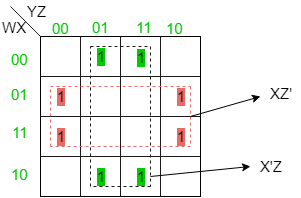ISRO | ISRO CS 2009 | Question 20

• Last Updated : 20 Nov, 2018

Consider the following boolean function of four variables,
f (w, x, y,z) = Σ(1, 3, 4, 6, 9, 11, 12, 14], the function is
(A) Independent of one variable
(B) Independent of two variables
(C) Independent of three variables
(D) Dependent on all variables

Explanation: The K-Map for the boolean function of four variables can be drawn as:So, independent of two variables.

Option (B) is correct.

Quiz of this Question

My Personal Notes arrow_drop_up